# ML Aggarwal Class 10 Solutions for ICSE Maths Chapter 22 Probability Ex 22

## ML Aggarwal Class 10 Solutions for ICSE Maths Chapter 22 Probability Ex 22

ML Aggarwal Class 10 Solutions for ICSE Maths Chapter 22 Probability Ex 22

Question 1.
A bag contains a red ball, a blue ball and a yellow ball, all the balls being of the same size. Anjali takes out a ball from the bag without looking into it. What is the probability that she takes out
(i) yellow ball?
(ii) red ball?
(iii) blue ball?
Solution:Question 2.
A box contains 600 screws, one-tenth are rusted. One screw is taken out at random from this box. Find the probability that it is a good screw.
Solution: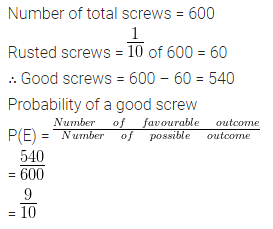Question 3.
In a lottery, there are 5 prized tickets and 995 blank tickets. A person buys a lottery ticket. Find the probability of his winning a prize.
Solution:Question 4.
12 defective pens are accidentally mixed with 132 good ones. It is not possible to just look at a pen and tell whether or not it is defective. One pen is taken out at random from this lot. Determine the probability that the pen is taken out is a good one.
Solution:Question 5.
If the probability of winning a game is $$\\ \frac { 5 }{ 11 }$$, what is the probability of losing ?
Solution:Question 6.
Two players, Sania and Sonali play a tennis match. It is known that the probability of Sania winning the match is 0.69. What is the probability of Sonali winning?
Solution:Question 7.
A bag contains 3 red balls and 5 black balls. A ball is drawn at random’ from in the bag. What is the probability that the ball drawn is?
(i) red?
(ii) not red?
Solution:Question 8.
There are 40 students in Class X of a school of which 25 are girls and the.others are boys. The class teacher has to select one student as a class representative. She writes the name of each student on a separate card, the cards being identical. Then she puts cards in a bag and stirs them thoroughly. She then draws one card from the bag. What is the probability that the name is written on the card is the name of
(i) a girl?
(ii) a boy?
Solution:Question 9.
A letter is chosen from the word ‘TRIANGLE’. What is the probability that it is a vowel?
Solution:Question 10.
A letter of the English alphabet is chosen at random. Determine the probability that the letter is a consonant.
Solution: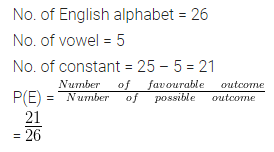Question 11.
A bag contains 5 black, 7 red and 3 white balls. A ball is drawn at random from the bag, find the probability that the ball drawn is:
(i) red
(ii) black or white
(iii) not black.
Solution:Question 12.
A box contains 7 blue, 8 white and 5 black marbles. If a marble is drawn at random from the box, what is the probability that it will be
(i) black?
(ii) blue or black?
(iii) not black?
(iv) green?
Solution: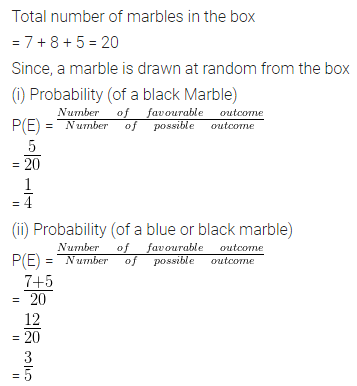Question 13.
A bag contains 6 red balls, 8 white balls, 5 green balls and 3 black balls. One ball is drawn at random from the bag. Find the probability that the ball is :
(i) white
(ii) red or black
(iii) not green
(iv) neither white nor black.
Solution: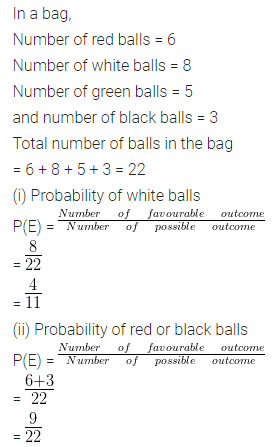Question 14.
A piggy bank contains hundred 50 p coins, fifty Rs 1 coins, twenty Rs 2 coins and ten Rs 5 coins. It is equally likely that one of the coins will fall down when the bank is turned upside down, what is the probability that the coin
(i) will be a 50 p coin?
(ii) will not be Rs 5 coin?
Solution:Question 15.
A carton consists of 100 shirts of which 88 are good, 8 have minor defects and 4 have major defects. Peter, a trader, will only accept the shirts which are good, but Salim, another trader, will only reject the shirts which have major defects. One shirt is drawn at random from the carton. What is the probability that
(i) it is acceptable to Peter?
(ii) it is acceptable to Salim?
Solution: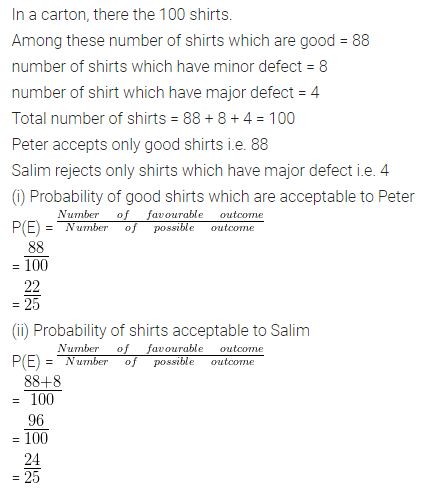Question 16.
A die is thrown once. What is the probability that the
(i) number is even
(ii) the number is greater than 2?
Solution:Question 17.
In a single throw of a die, find the probability of getting:
(i) an odd number
(ii) a number less than 5
(iii) a number greater than 5
(iv) a prime number
(v) a number less than 8
(vi) a number divisible by 3
(vii) a number between 3 and 6
(viii) a number divisible by 2 or 3.
Solution: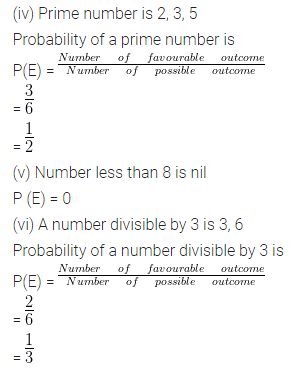Question 18.
A die has 6 faces marked by the given numbers as shown below: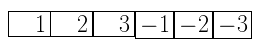The die is thrown once. What is the probability of getting
(i) a positive integer.
(ii) an integer greater than – 3.
(iii) the smallest integer ?
Solution: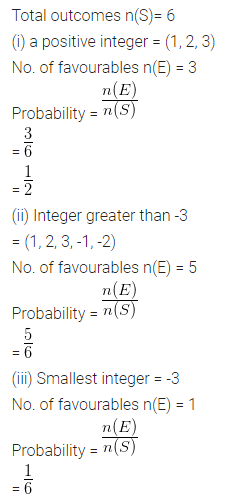Question 19.
A game of chance consists of spinning an arrow which comes to rest pointing at one of the numbers 1, 2, 3, 4, 5, 6, 7, 8 (shown in the adjoining figure) and these are equally likely outcomes. What is the probability that it will point at
(i) 8?
(ii) an odd number?
(iii) a number greater than 2?
(iv) a number less than 9?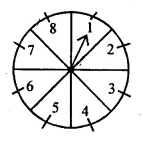Solution:Question 20.
Find the probability that the month of January may have 5 Mondays in
(i) a leap year
(ii) a non-leap year.
Solution:Question 21.
Find the probability that the month of February may have 5 Wednesdays in
(i) a leap year
(ii) a non-leap year.
Solution: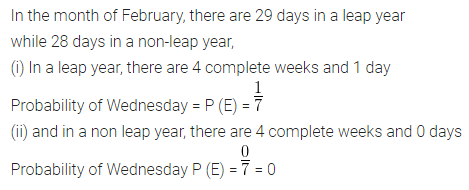Question 22.
Sixteen cards are labelled as a, b, c,…, m, n, o, p. They are put in a box and shuffled. A boy is asked to draw a card from the box. What is the probability that the card drawn is:
(i) a vowel
(ii) a consonant
(iii) none of the letters of the word median.
Solution:Question 23.
An integer is chosen between 0 and 100. What is the probability that it is
(i) divisible by 7?
(ii) not divisible by 7?
Solution:Question 24.
Cards marked with numbers 1, 2, 3, 4, 20 are well shuffled and a card is drawn at random.
What is the probability that the number on the card is
(i) a prime number
(ii) divisible by 3
(iii) a perfect square? (2010)
Solution:Question 25.
A box contains 25 cards numbered 1 to 25. A card is drawn from the box at random. Find the probability that the number on the card is :
(i) even
(ii) prime
(iii) multiple of 6
Solution: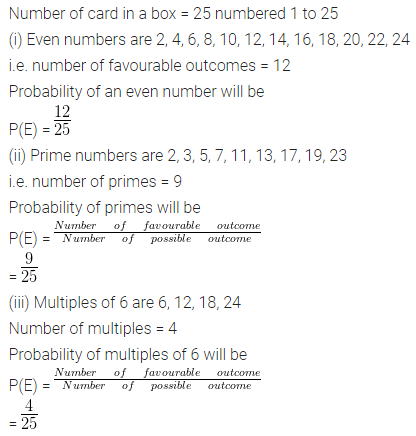Question 26.
A box contains 15 cards numbered 1, 2, 3,…..15 which are mixed thoroughly. A card is drawn from the box at random. Find the probability that the number on the card is :
(i) Odd
(ii) prime
(iii) divisible by 3
(iv) divisible by 3 and 2 both
(v) divisible by 3 or 2
(vi) a perfect square number.
Solution: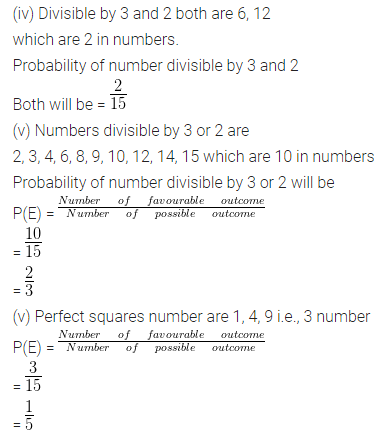Question 27.
A box contains 19 balls bearing numbers 1, 2, 3,…., 19. A ball is drawn at random
from the box. Find the probability that the number on the ball is :
(i) a prime number
(ii) divisible by 3 or 5
(iii) neither divisible by 5 nor by 10
(iv) an even number.
Solution: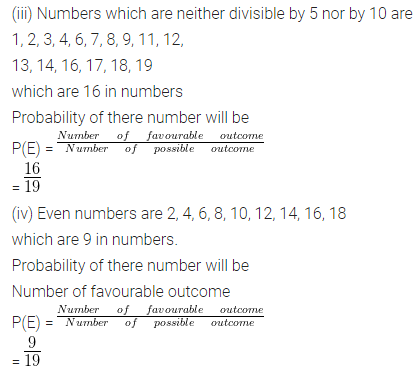Question 28.
Cards marked with numbers 13, 14, 15, …, 60 are placed in a box and mixed thoroughly. One card is drawn at random from the box. Find the probability that the number on the card drawn is
(i) divisible by 5
(ii) a perfect square number.
Solution:Question 29.
Tickets numbered 3, 5, 7, 9,…., 29 are placed in a box and mixed thoroughly. One ticket is drawn at random from the box. Find the probability that the number on the ticket is
(i) a prime number
(ii) a number less than 16
(iii) a number divisible by 3.
Solution:Question 30.
A box contains 90 discs which are numbered from 1 to 90. If one disc is drawn at random from the box, find the probability that it bears
(i) a two-digit number
(ii) a perfect square number
(iii) a number divisible by 5.
Solution:Question 31.
Cards marked with numbers 2 to 101 are placed in a box and mixed thoroughly. One card is drawn at random from this box. Find the probability that the number on the card is
(i) an even number
(ii) a number less than 14
(iii) a number which is a perfect square
(iv) a prime number less than 30.
Solution:Question 32.
A bag contains 15 balls of which some are white and others are red. If the probability of drawing a red ball is twice that of a white ball, find the number of white balls in the bag.
Solution:Question 33.
A bag contains 6 red balls and some blue balls. If the probability of drawing a blue ball is twice that of a red ball, find the number of balls in the bag.
Solution:Question 34.
A bag contains 24 balls of which x are red, 2x are white and 3x are blue. A blue is selected at random. Find the probability that it is
(i) white
(ii) not red.
Solution: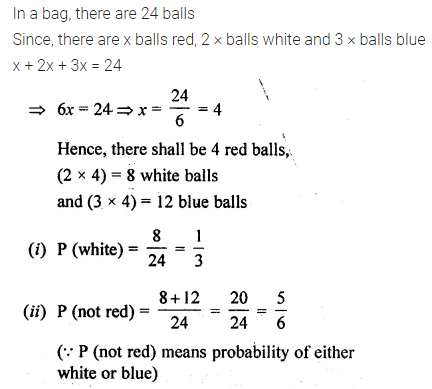Question 35.
A card is drawn from a well-shuffled pack of 52 cards. Find the probability of getting:
(ii) a jack.
(iii) a king of red colour
(iv) a card of diamond
(v) a king or a queen
(vi) a non-face card
(vii) a black face card
(viii) a black card
(ix) a non-ace
(x) non-face card of black colour
(xi) neither a spade nor a jack
(xii) neither a heart nor a red king
Solution: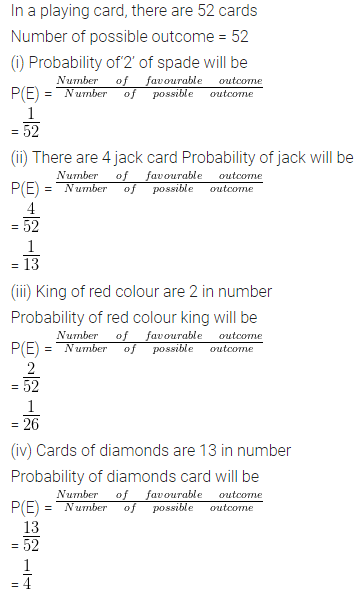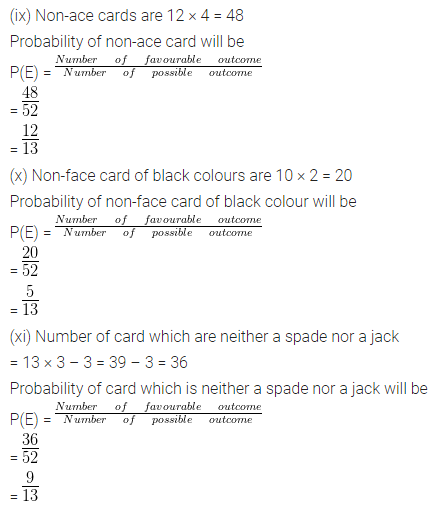Question 36.
All the three face cards of spades are removed from a well-shuffled pack of 52 cards. A card is then drawn at random from the remaining pack. Find the probability of getting
(i) a black face card
(ii) a queen
(iii) a black card
(iv) a heart
(vi) ‘9’ of black colour
Solution:Question 37.
From a pack of 52 cards, a blackjack, a red queen and two black kings fell down. A card was then drawn from the remaining pack at random. Find the probability that the card drawn is
(i) a black card
(ii) a king
(iii) a red queen.
Solution: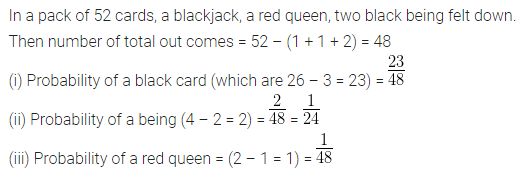Question 38.
Two coins are tossed once. Find the probability of getting:
(ii) at least one tail.
Solution:Question 39.
Two different coins are tossed simultaneously. Find the probability of getting :
(i) two tails
(ii) one tail
(iii) no tail
(iv) at most one tail.
Solution:Question 40.
Two different dice are thrown simultaneously. Find the probability of getting:
(i) a number greater than 3 on each dice
(ii) an odd number on both dice.
Solution: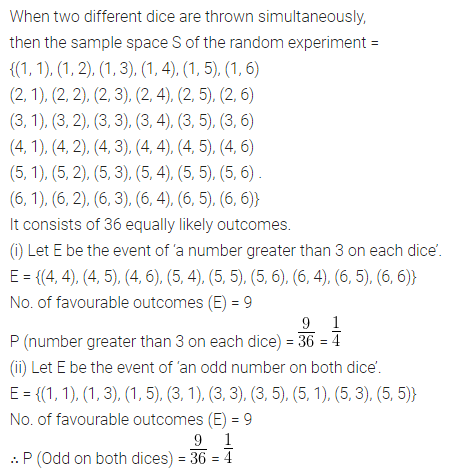Question 41.
Two different dice are thrown at the same time. Find the probability of getting :
(i) a doublet
(ii) a sum of 8
(iii) sum divisible by 5
(iv) the sum of at least 11.
Solution: